Home MonkeyNotes Printable Notes Digital Library Study Guides Study Smart Parents Tips College Planning Test Prep Fun Zone Help / FAQ How to Cite New Title Request

4.8 Derivative of A Composite Function

Definition :

If f and g are two functions defined by y = f(u) and u = g(x) respectively then a function defined by y = f [g(x)] or fog(x) is called a composite function or a function of a function.

The theorem for finding the derivative of a composite function is known as the CHAIN RULE.

Theorem :

If f and g are differentiable and are defined by y = f (u) and u = g (x), then the composite function y = f [ g (x) ] is differentiable and we have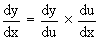Corollary :

If y = f (u), u = g (v) and v = h (x) where f, g and h are differentiable functions of u, v and x respectively, then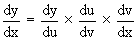Example 18

Show that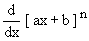=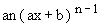Your browser does not support the IFRAME tag.

Solution :

Let y =and u = ax + b

\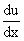= a

Then,

y = un

\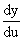= nun-1= n(ax + b) n-1

Now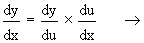by chain rule.

\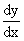= n(ax + b) n-1  x  a

= an(ax + b) n-1

Example 19

Findif y = (2x3  5x2 + 4)5

Solution :

Let y = u5

and u = 2x3  5x2 + 4

\= 6x2  10x

\= 5u4

Nowby chain rule.

\Index

4. 1 Derivability At A Point
4. 2 Derivability In An Interval
4. 3 Derivability And Continuity Of A Function At A Point
4. 4 Some Counter Examples
4. 5 Interpretation Of Derivatives
4. 6 Theorems On Derivatives (differentiation Rules)
4. 7 Derivatives Of Standard Functions
4. 8 Derivative Of A Composite Function
4. 9 Differentiation Of Implicit Functions
4.10 Derivative Of An Inverse Function
4.11 Derivatives Of Inverse Trigonometric Functions
4.12 Derivatives Of Exponential & Logarithmic Functions
4.13 Logarithmic Differentiation
4.14 Derivatives Of Functions In Parametric Form
4.15 Higher order Derivatives

Chapter 5